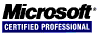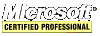## Working with Arrays in ASP.NET

.NET makes working with arrays incredibly easy. One can sort an array or two using the static method Array.Sort().

To sort the following 10,000,000 element random array takes 00:00:01.4900021 to finish. Probably, QuickSort is being used because this algorithm works very well on completely random arrays and does not have a time penalty to sort an already sorted array. To confirm this, a 10,000,000 element random array was quicksorted in 1.28 seconds using C/C++ (on the same machine).

``````Random r = new Random();
int[] ai = new int;
for (int i = 0; i < 10000000; ai[i++] = r.Next()) ;
Array.Sort(ai);``````

Sort two arrays at the same time!

``````int[] aNum = { 2, 0, 1 };
string[] aStr = { "Arizona", "California", "Texas" };
Array.Sort(aNum, aStr);``````

Now, `aStr[]` is arranged as "California" "Texas" and "Arizona" to match `aNum[]` which is sorted as 0, 1 and 2. This is an example of parallel arrays.

There is a brief arrays tutorial here msdn.microsoft.com.